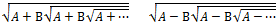# Nested Square Root CalculatorA =      B =
+

A nested radical is an expression of the form

sqrt(a₁ ± b₁⋅sqrt(a₂ ± b₂⋅sqrt(a₃ ± ...)))

Even if the square roots are infinitely nested, the expression will converge to a real number if the coefficients ak and bk satisfy certain conditions.

If all of the ak's are equal to some constant A, and all of the bk's are equal to some constant B, then you can easily solve for the convergent value by setting up and solving a quadratic equation.

You can use the calculator above to compute the exact values of infinitely nested square roots with constant coefficients, or follow the examples below to figure their values by hand.

If x = sqrt(A + B⋅sqrt(A + B⋅sqrt(A + B⋅sqrt(A + B⋅sqrt(A + ..., then squaring both sides yields the equivalent expression

x² = A + B⋅sqrt(A + B⋅sqrt(A + B⋅sqrt(A + ...

And this is equivalent to

x² = A + Bx.

By rearranging the equation into x² - Bx - A = 0, you can use the quadratic formula to find the exact value of x:

x = B/2 + sqrt(B²/4 + A).

Similarly, if y = sqrt(A − B⋅sqrt(A − B⋅sqrt(A − ..., then the exact value of y is given by

y = -B/2 + sqrt(B²/4 + A).

### Example 1

Every positive number n can be represented by an infinitely nested radical. For example, if the operators are all "+" with B = 1 and A = n(n-1), then

n = sqrt[n(n-1) + sqrt[n(n-1) + sqrt[n(n-1) + ...

Similarly, if the operators are "−" with B = 1 and A = n(n+1), then

n = sqrt[n(n+1) - sqrt[n(n+1) - sqrt[n(n+1) - ...

These constructions give two ways of representing the number 4:

4 = sqrt(12 + sqrt(12 + sqrt(12 + sqrt(12 + ...            and

4 = sqrt(20 − sqrt(20 − sqrt(20 − sqrt(20 − ...

### More Examples

To evaluate the nested square root

sqrt(4 + 3⋅sqrt(4 + 3⋅sqrt(4 + 3⋅sqrt(4 + ...

we set A = 4 and B = 3 and use the first formula. This gives us

3/2 + sqrt(9/4 + 4)
= 1.5 + 2.5
= 4

which provides another nested radical expression for the number 4.

The golden ratio φ = (1+√5)/2 = 1.6180339887... can also be represented by an infinitely nested square root by setting A = B = 1.

φ = sqrt(1 + sqrt(1 + sqrt(1 + sqrt(1 + ...

The exact value of some infinitely nested radicals cannot be computed by solving a quadratic equation. In the expression

sqrt(1 + sqrt(2 + sqrt(3 + sqrt(4 + sqrt(5 + sqrt(6 + sqrt(7 + ...

the ak's grow linearly, thus the radical cannot be simplified using the quadratic method above. The value of this expression is known as the nested radical constant and is equal to

1.7579327566180045327088196382181385276531999221468377043101355...

However, there are some infinitely nested radicals with non-constant values of ak and bk that can be simplified. Consider the number

p = sqrt(1 + 2⋅sqrt(1 + 3⋅sqrt(1 + 4⋅sqrt(1 + 5⋅sqrt(1 + 6⋅sqrt(1 + 7⋅sqrt(1 + ...

If we consider a function F(x) defined as

F(x) = sqrt(1 + (x+1)⋅sqrt(1 + (x+2)⋅sqrt(1 + (x+3)⋅sqrt(1 + (x+4)⋅sqrt(1 + ...

then the value of p is equal to F(1). By squaring both sides we get the functional relation

F(x)² = 1 + (x+1)F(x+1)

The solution to this equation is the linear function F(x) = x + 2. Thus, the value of p is F(1) = 1 + 2 = 3. Using a very similar argument, you can show that

3 = sqrt(4 + 1⋅sqrt(4 + 3⋅sqrt(4 + 5⋅sqrt(4 + 7⋅sqrt(4 + 9⋅sqrt(4 + ...